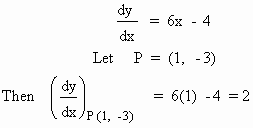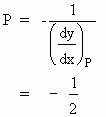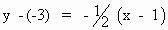Home MonkeyNotes Printable Notes Digital Library Study Guides Study Smart Parents Tips College Planning Test Prep Fun Zone Help / FAQ How to Cite New Title Request

Example 1

Find the equation of the tangent and normal to the curve y = 3x2 - 4x -2 at the point (1, -3)

Solution : y = 3x2 - 4x -2 ® The curve.

By Differentiating w. r. to x, we get\ Equation of the tangent at point P (1, -3) is

y - (-3) = 2 (x - 1)

i.e. y + 3 = 2x -2

i.e. y = 2x -5Your browser does not support the IFRAME tag.

Next, slope of the normal at\ Equation of the normal at P (1, -3) isi.e. 2 (y + 3) = - (x - 1)

i.e. x + 2y + 5 = 0

Index

5.1 Tangent And Normal Lines
5.2 Angle Between Two Curves
5.3 Interpretation Of The Sign Of The Derivative
5.4 Locality Increasing Or Decreasing Functions 5.5 Critical Points
5.6 Turning Points
5.7 Extreme Value Theorem
5.8 The Mean-value Theorem
5.9 First Derivative Test For Local Extrema
5.10 Second Derivative Test For Local Extrema
5.11 Stationary Points
5.12 Concavity And Points Of Inflection
5.13 Rate Measure (distance, Velocity And Acceleration)
5.14 Related Rates
5.15 Differentials : Errors And ApproximationAll Contents Copyright © All rights reserved.
Further Distribution Is Strictly Prohibited.

1209688 PinkMonkey users are on the site and studying right now.